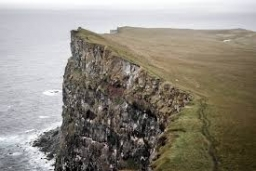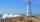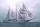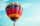# Depth angle

From a cliff of 150 meters high, we can see the ship at a depth angle of 9° at sea. How far is the ship from the cliff?

x =  947.0627 m

### Step-by-step explanation:We will be pleased if You send us any improvements to this math problem. Thank you!Tips to related online calculators
Do you want to convert length units?

#### You need to know the following knowledge to solve this word math problem:

We encourage you to watch this tutorial video on this math problem:

## Related math problems and questions:

• Observation towerFrom the observation tower at a height of 105 m above sea level, the ship is aimed at a depth angle of 1° 49´. How far is the ship from the base of the tower?
• Powerplant chimneyFrom the building window at the height of 7.5 m, we can see the top of the factory chimney at an altitude angle of 76° 30 ′. We can see the chimney base from the same place at a depth angle of 5° 50 ′. How tall is the chimney?
• The rescue helicopterThe rescue helicopter is above the landing site at a height of 180m. The site of the rescue operation can be seen from here at a depth angle of 52° 40 '. How far will the helicopter land from the rescue site?
• How farFrom the top of a lighthouse 145 ft above sea level, the angle of depression of a boat 29°. How far is the boat from the lighthouse?
• TreeBetween points A and B is 50m. From A we see a tree at an angle 18°. From point B we see the tree in three times bigger angle. How tall is a tree?
• Depth anglesAt the top of the mountain stands a castle, which has a tower 30 meters high. We see the crossroad in the valley from the top of the tower and heel at depth angles of 32° 50 'and 30° 10'. How high is the top of the mountain above the crossroad
• Elevation anglesFrom the endpoints of the base 240 m long and inclined at an angle of 18° 15 ', the top of the mountain can be seen at elevation angles of 43° and 51°. How high is the mountain?
• Balloon and bridgeFrom the balloon, which is 92 m above the bridge, one end of the bridge is seen at a depth angle of 37° and the second end at depth angle 30° 30 '. Calculate the length of the bridge.
• SteepleWe see the church tower from the road at an angle of 52°. When we zoom out to 29 meters away, it can be seen at an angle of 21°. How high is it?
• The mastThe top of the pole we see at an angle of 45°. If we approach the pole by 10 m, we see the top of the pole at an angle of 60°. What is the height of the pole?
• AircraftThe plane flies at altitude 6500 m. At the time of first measurement was to see the elevation angle of 21° and second measurement of the elevation angle of 46°. Calculate the distance the plane flew between the two measurements.
• Two boatsTwo boats are located from a height of 150m above the surface of the lake at depth angles of 57° and 39°. Find the distance of both boats if the sighting device and both ships are in a plane perpendicular to the surface of the lake.
• SeaHow far can you see from the ship's mast, whose peak is at 14 meters above sea level? (Earth's radius is 6370 km).
• BuildingHow high is the building that throws horizontal shadow 95.4 m long at angle 50°?
• Altitude angleIn complete winds-free weather, the balloon took off and remained standing exactly above the place from which it took off. It is 250 meters away from us. How high did the balloon fly when we see it at an altitude angle of 25°?
• CloudsApproximately at what height is the cloud we see under an angle of 26°10' and see the Sun at an angle of 29°15' and the shade of the cloud is 92 meters away from us?
• Tower's viewFrom the church tower's view at the height of 65 m, the top of the house can be seen at a depth angle of alpha = 45° and its bottom at a depth angle of beta = 58°. Calculate the height of the house and its distance from the church.